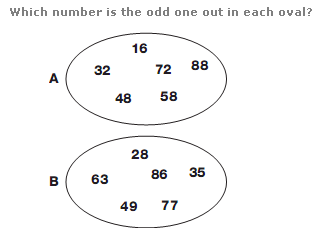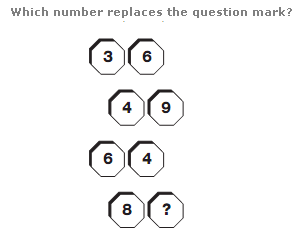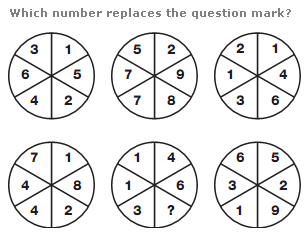# Puzzles - Number puzzles

### Exercise :: Number puzzlesAnswer : A:58   B:86 Explanation : In the first oval, all numbers are multiples of 8, and in the second, they are all multiples of 7.Answer : 1 Explanation : Reading each pair of numbers as a 2 digit number, they follow the sequence of square numbers from 6 to 9.Answer : 7 Explanation : Taking the top row of circles, numbers in the central circle equal the sum of the numbers in corresponding segments of the left and right hand circles. In the bottom row, numbers in the central circle equal the difference between numbers in corresponding segments of the left and right hand circles.